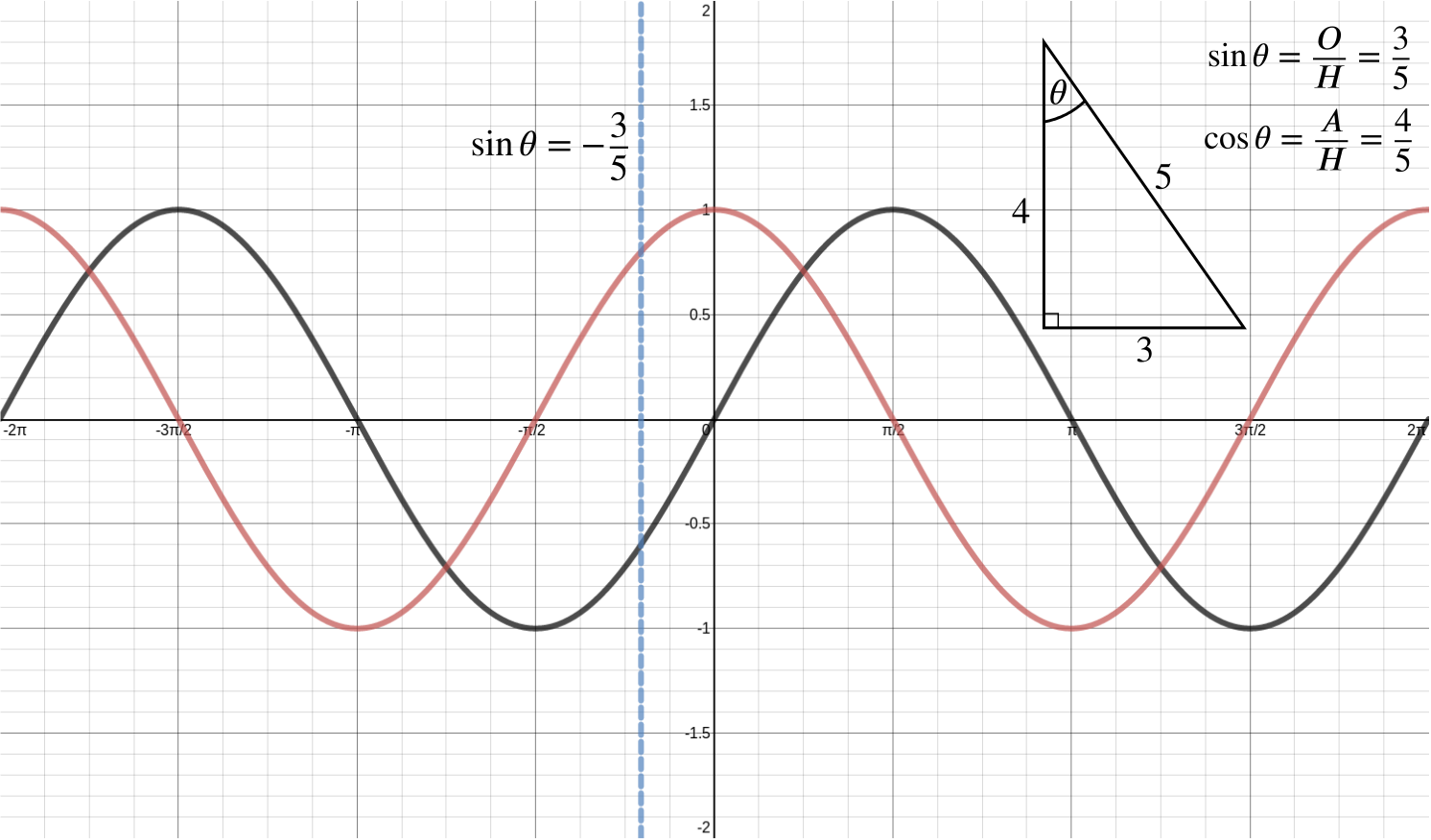Review question

# Can we find where the normal is parallel to $y=x$? Add to your resource collection Remove from your resource collection Add notes to this resource View your notes for this resource

Ref: R8939

## Solution

A curve has parametric equations $x=5a\sec\theta,\,y=3a\tan\theta$, where $-\frac{1}{2}\pi < \theta < \frac{1}{2}\pi$ and $a$ is a positive constant. Find the coordinates of the point on the curve at which the normal is parallel to the line $y=x$.

Differentiating with respect to $\theta$, we find that \begin{align*} \frac{dx}{d\theta} &= 5a\sec\theta\tan\theta \\ \frac{dy}{d\theta} &= 3a\sec^2\theta . \end{align*}

Thus $\frac{dy}{dx}=\frac{3a\sec^2\theta}{5a\sec\theta\tan\theta}=\frac{3}{5\sin\theta}.$

The normal to the curve therefore has gradient $-\frac{5}{3}\sin\theta.$

The normal is parallel to $y=x$ when $-\frac{5}{3}\sin\theta=1\quad \Longrightarrow \quad \sin\theta=-\frac{3}{5}.$

By sketching a graph of $y=\sin\theta$, we can see that there’s a unique value of $\theta$ in the range $-\dfrac{\pi}{2}<\theta<\dfrac{\pi}{2}$ for which $\sin\theta=-\dfrac{3}{5}$.

The blue line in the diagram shows that this corresponds to a positive value of $\cos\theta$.

Hence, we get that $\cos^2 \theta = 1 - \sin^2 \theta = 1 - \left(-\frac{3}{5}\right)^2 = \frac{16}{25} \quad \Longrightarrow \quad \cos \theta = \frac{4}{5}$ as $\cos\theta$ is positive.$y=\sin\theta$ (red), $y=\cos\theta$ (black) and the value of $\theta$ for which the normal is parallel to $y=x$ (blue)

Alternatively, using the sketch above, we can see that we are dealing with a $3-4-5$ triangle, and so the value of cosine we need is $\cos\theta=\dfrac{4}{5}$.

Thus our coordinates for this value of $\theta$ are \begin{align*} x &= 5a\sec\theta = \frac{5a}{\cos\theta} = \frac{5a\times 5}{4} = \frac{25a}{4}, \\ y &= 3a\tan\theta=3a \times \frac{\sin \theta}{\cos \theta} = 3a\times\left(-\frac{3}{5}\right)\times\left(\frac{5}{4}\right) = -\frac{9a}{4}. \end{align*}